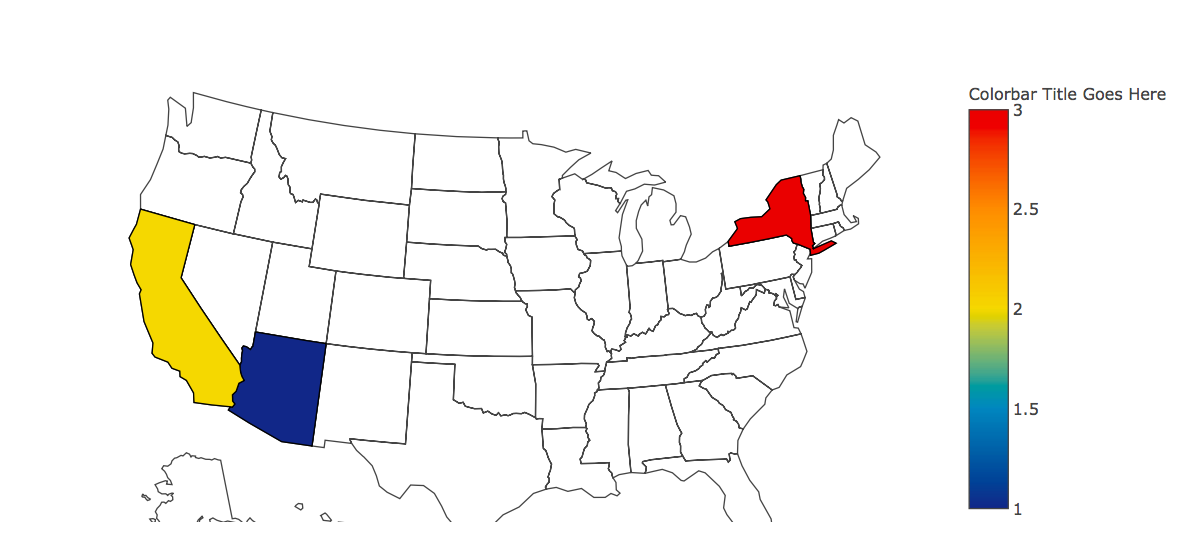# Python | Geographical plotting using plotly

Geographical plotting is used for world map as well as states under a country. Mainly used by data analysts to check the agriculture exports or to visualize such data.

plotly is a Python library which is used to design graphs, especially interactive graphs. It can plot various graphs and charts like histogram, barplot, boxplot, spreadplot and many more. It is mainly used in data analysis as well as financial analysis. plotly is an interactive visualization library.

cufflink connects plotly with pandas to create graphs and charts of dataframes directly. choropleth is used to describe geographical plotting of USA. choropleth is used in the plotting of world maps and many more.

Command to install plotly:

`pip install plotly `

Below is the implementation:

 `# Python program to plot  ` `# geographical data using plotly ` ` `  `# importing all necessary libraries ` `import` `plotly.plotly as py ` `import` `plotly.graph_objs as go ` `import` `pandas as pd ` ` `  `# some more libraries to plot graph ` `from` `plotly.offline ``import` `download_plotlyjs, init_notebook_mode, iplot, plot ` ` `  `# To establish connection ` `init_notebook_mode(connected ``=` `True``) ` ` `  ` `  `# type defined is choropleth to ` `# plot geographical plots ` `data ``=` `dict``(``type` `=` `'choropleth'``, ` ` `  `            ``# location: Arizoana, California, Newyork ` `            ``locations ``=` `[``'AZ'``, ``'CA'``, ``'NY'``], ` `             `  `            ``# States of USA ` `            ``locationmode ``=` `'USA-states'``, ` `             `  `            ``# colorscale can be added as per requirement ` `            ``colorscale ``=` `'Portland'``, ` `             `  `            ``# text can be given anything you like ` `            ``text ``=` `[``'text 1'``, ``'text 2'``, ``'text 3'``], ` `            ``z ``=` `[``1.0``, ``2.0``, ``3.0``], ` `            ``colorbar ``=` `{``'title'``: ``'Colorbar Title Goes Here'``}) ` `             `  `layout ``=` `dict``(geo ``=``{``'scope'``: ``'usa'``}) ` ` `  `# passing data dictionary as a list  ` `choromap ``=` `go.Figure(data ``=` `[data], layout ``=` `layout) ` ` `  `# plotting graph ` `iplot(choromap) `

Output:My Personal Notes arrow_drop_upCheck out this Author's contributed articles.

If you like GeeksforGeeks and would like to contribute, you can also write an article using contribute.geeksforgeeks.org or mail your article to contribute@geeksforgeeks.org. See your article appearing on the GeeksforGeeks main page and help other Geeks.

Please Improve this article if you find anything incorrect by clicking on the "Improve Article" button below.

Article Tags :

1

Please write to us at contribute@geeksforgeeks.org to report any issue with the above content.